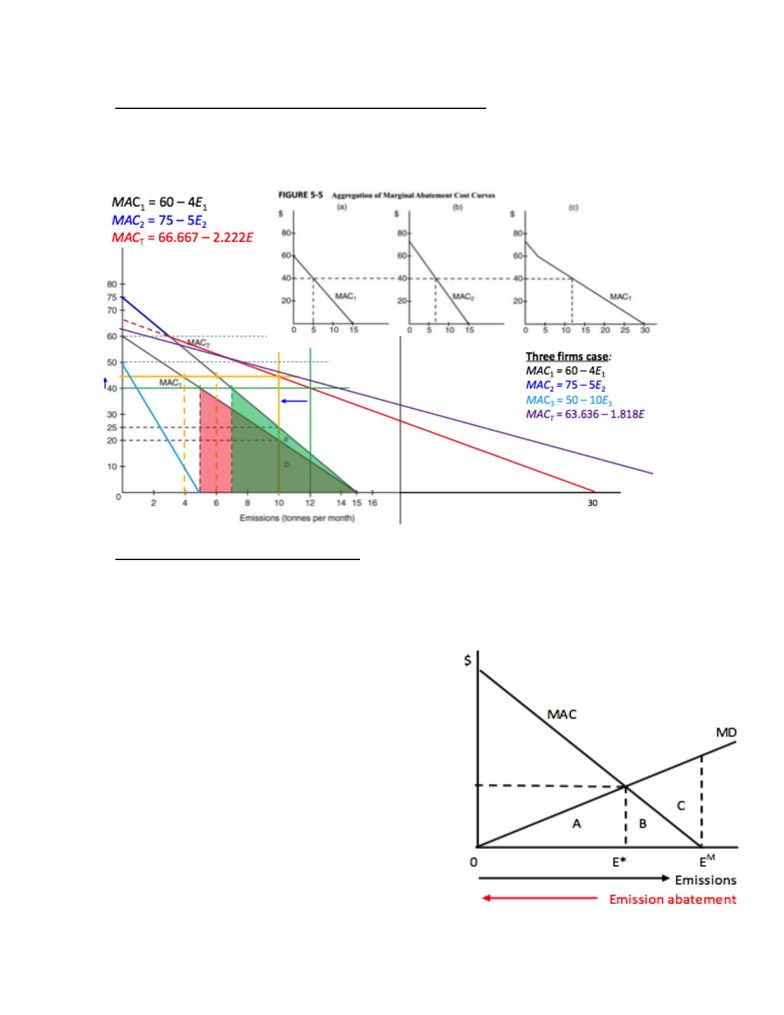# Chpt 5 Pt 3.docx

58 views3 pagesEfficent abatement using equal marginal principle- 2
The aggregate MAC cruve can be used to determine the efficient pollution
abtement by two or more firms as the aggregate MAC statisfies the qual
marginal principle
Socially efficient level of emissions
Socially efficient level of emissions is where the total social costs imposed on
society is minimized
Total social costs (TSC) = total damage costs (TDC) + total abatement costs
(TAC)
o TSC=TDC+TAC
Minimzied at point E* where MD=MAC
o AT E* MD=MAC, E decreases by
EM E*
TDC= A
TAC=B
TSC=A+B
o At EM no pollution ocntol, this is
competitive market level
emission
TDC= A+B+C
TAC= 0
TSCM= A+B+C
DWL for no control = C
Unlock document

This preview shows page 1 of the document.
Unlock all 3 pages and 3 million more documents.

# Get access

\$10 USD/m
Billed \$120 USD annually
Homework Help
Class Notes
Textbook Notes